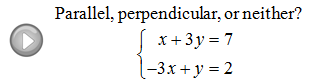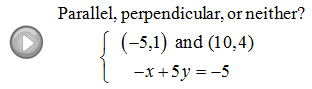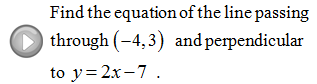## Algebra

### Parallel and Perpendicular Lines

Many of the problems that we will encounter in this section involve parallel or perpendicular lines.  To study this we must focus on the slopes of the lines.InteractiveParallel Lines ]

There is notation that corresponds to these ideas, for example,reads "m perpendicular".  To find the slope of the perpendicular line simply reciprocate the number and change the sign.

InteractivePerpendicular Lines ]

Find the corresponding perpendicular slope.Of course, just use the same slope if you are asked to find the slope a parallel line.

Are the lines parallel, perpendicular or neither?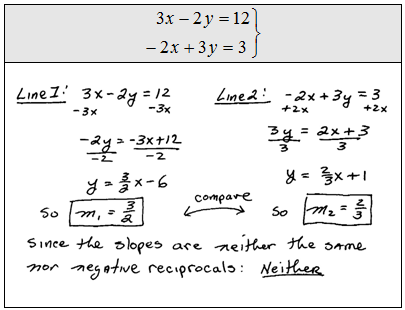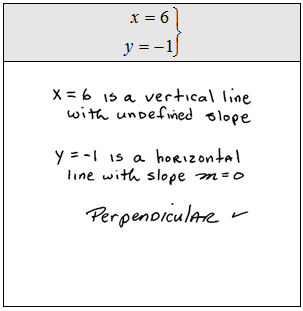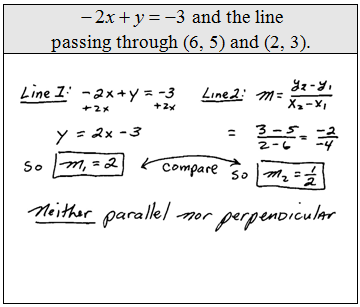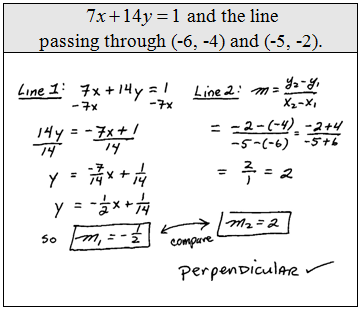Find the slope of the line perpendicular.Now we use these facts and the formula for equations of lines to find equations given certain geometric information. Recall,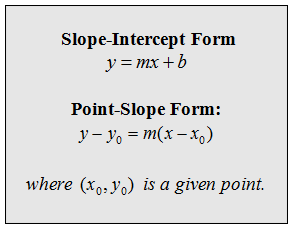Example: Find the equation of the line perpendicular to y = −1/4 x + 2 passing through the point (−1,−5).This three step process using slope-intercept form always works. We could find this equation using point-slope form as well as demonstrated below.

Example: Find the equation of the line perpendicular to y = −1/4 x + 2 passing through the point (−1, −5).Notice that the answer y = 4x − 1 is the same using either method.

Find the equation of the line. (Using slope-intercept form.)Find the equation of the line. (Using point-slope form.)